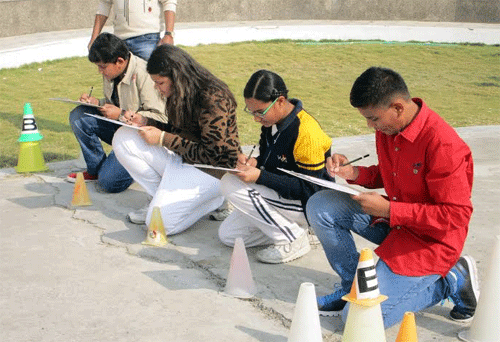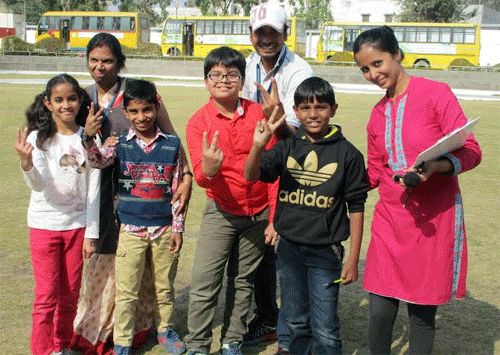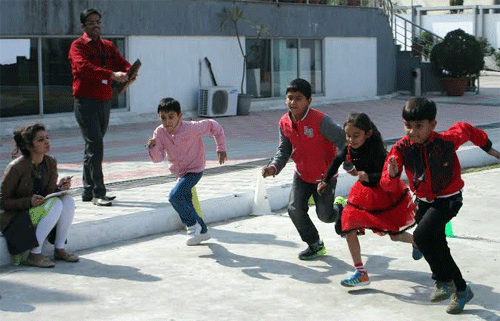# Wittians add interest & subtract fear of Mathematics

Wittians celebrated the Birthday of Srinivasa Ramanujan, Indian mathematician, who made extraordinary contributions to mathematical analysis, number theory, infinite series, and continued fractions in the most interesting way ever.Wittians celebrated the Birthday of Srinivasa Ramanujan, Indian mathematician, who made extraordinary contributions to mathematical analysis, number theory, infinite series, and continued fractions in the most interesting way ever.

A Mathematical Relay Race was conducted for students of Grade I to VIII wherein instead of exchanging batons students solved various mathematical problems at each point. It also served as the most interesting way of teaching mathematics and creating an interest for mathematics in students.Students participated in the relay race with great zeal and enthusiasm. Principal Shubha Govil gave the clapper for the race and also told the students about the importance of practical mathematics in life.